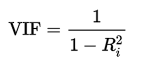# Variance Inflation Factor

Share on

Statistics Definitions > Variance Inflation Factor

## What is a Variance Inflation Factor?

A variance inflation factor(VIF) detects multicollinearity in regression analysis. Multicollinearity is when there’s correlation between predictors (i.e. independent variables) in a model; it’s presence can adversely affect your regression results. The VIF estimates how much the variance of a regression coefficient is inflated due to multicollinearity in the model.

VIFs are usually calculated by software, as part of regression analysis. You’ll see a VIF column as part of the output. VIFs are calculated by taking a predictor, and regressing it against every other predictor in the model. This gives you the R-squared values, which can then be plugged into the VIF formula. “i” is the predictor you’re looking at (e.g. x1 or x2):## Interpreting the Variance Inflation Factor

Variance inflation factors range from 1 upwards. The numerical value for VIF tells you (in decimal form) what percentage the variance (i.e. the standard error squared) is inflated for each coefficient. For example, a VIF of 1.9 tells you that the variance of a particular coefficient is 90% bigger than what you would expect if there was no multicollinearity — if there was no correlation with other predictors.
A rule of thumb for interpreting the variance inflation factor:

• 1 = not correlated.
• Between 1 and 5 = moderately correlated.
• Greater than 5 = highly correlated.

Exactly how large a VIF has to be before it causes issues is a subject of debate. What is known is that the more your VIF increases, the less reliable your regression results are going to be. In general, a VIF above 10 indicates high correlation and is cause for concern. Some authors suggest a more conservative level of 2.5 or above.

Sometimes a high VIF is no cause for concern at all. For example, you can get a high VIF by including products or powers from other variables in your regression, like x and x2. If you have high VIFs for dummy variables representing nominal variables with three or more categories, those are usually not a problem.

## References

Dodge, Y. (2008). The Concise Encyclopedia of Statistics. Springer.
Everitt, B. S.; Skrondal, A. (2010), The Cambridge Dictionary of Statistics, Cambridge University Press.

CITE THIS AS:
Stephanie Glen. "Variance Inflation Factor" From StatisticsHowTo.com: Elementary Statistics for the rest of us! https://www.statisticshowto.com/variance-inflation-factor/
---------------------------------------------------------------------------Need help with a homework or test question? With Chegg Study, you can get step-by-step solutions to your questions from an expert in the field. Your first 30 minutes with a Chegg tutor is free!